# Polynomial Regression using Turicreate

• Difficulty Level : Medium
• Last Updated : 24 Jan, 2021

In this article, we will discuss the implementation of Polynomial Regression using Turicreate. Polynomial Regression: Polynomial regression is a form of regression analysis that models the relationship between a dependent say y and an independent variable say x as a nth degree polynomial. It is expressed as :

y= b0+b1x1+ b2x12+ b2x13+…… bnx1n

[where b0, b1, b2, …… bn are regression coefficients]

So let’s learn this concept through practicals.

Step 1: Import the important libraries and generate a very small data set using SArray and SFrame in turicreate that we are going to use to perform Polynomial Regression.

## Python3

 `# importing required python libraries``import` `turicreate``import` `matplotlib.pyplot as plt``import` `random`` ` `# Generating datapoints``X ``=` `[data ``for` `data ``in` `range``(``1``, ``21``)]``Y ``=` `[random.randrange(``100``, ``1000``, ``1``) ``for` `data ``in` `range``(``20``)]`` ` `# Creating Sarrays from the generated data points``Xs ``=` `turicreate.SArray(X, dtype``=``float``)``Ys ``=` `turicreate.SArray(Y, dtype``=``float``)`` ` `print``(f``"""Xs : {Xs}``\n-------------------------------------------------------------------------------------------\n``Ys : {Ys}"""``)`

Output: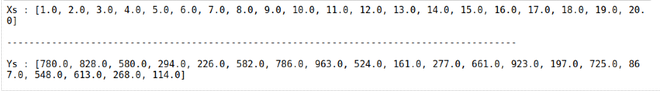Step 2: Plotting the generated data

## Python3

 `# plotting the generated data``plt.scatter(Xs, Ys)``plt.show()`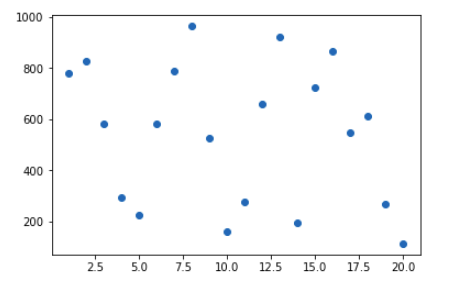Step 3: Create an SFrame containing the input, its polynomial_degrees, and the output in order to fit our regression model.

## Python3

 `# Creating an Sframe where all the inputs and the polynomial degree and output``def` `createSframe(inputs, pol_degree):``    ``datapoints ``=` `turicreate.SFrame({``'x1'``: inputs})``    ``for` `degree ``in` `range``(``2``, pol_degree``+``1``):``        ``datapoints[f``'x{degree}'``] ``=` `datapoints[f``'x{degree-1}'``]``*``datapoints[``'x1'``]``    ``return` `datapoints`` ` ` ` `# Creating a SFrame with polynomial degree 20``data_points ``=` `createSframe(Xs, ``20``)``data_points[``'y'``] ``=` `Ys`` ` `# showing the first 10 entries in the SFrame``data_points.head()`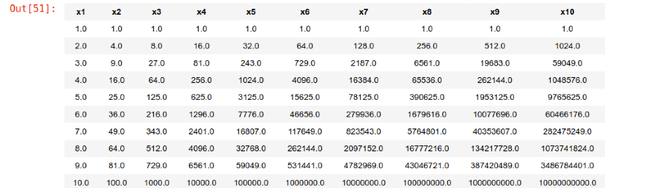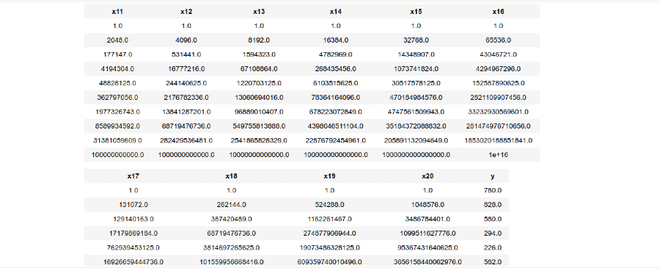Step 4: Fitting Polynomial Regression to the generated Data set.

## Python3

 `# Polynomial Regression``features ``=` `[f``'x{i}'` `for` `i ``in` `range``(``1``, ``21``)]``poly_model ``=` `turicreate.linear_regression.create(``    ``data_points, features``=``features, target``=``'y'``)`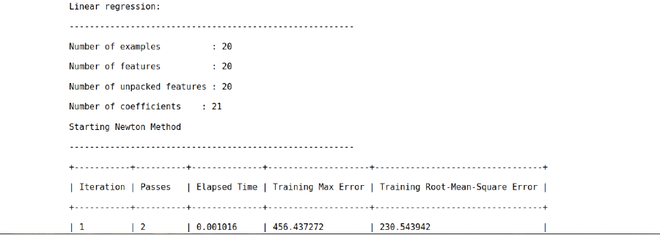Step 5: Predicting the result using the fitted model and storing the result in the SFrame.

## Python3

 `# predicting the some data``# Generating test datapoints``test_X ``=` `[random.randrange(``1``, ``60``, ``1``) ``for` `data ``in` `range``(``20``)]``test_Xs ``=` `turicreate.SArray(X, dtype``=``float``)``test_data ``=` `createSframe(test_Xs, ``5``)``data_points[``'predicted_y'``] ``=` `poly_model.predict(test_data)`` ` `data_points.head()`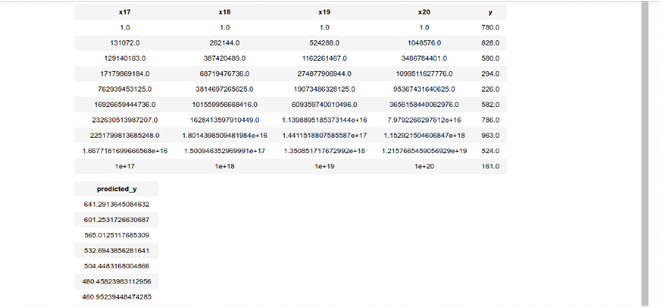Step 6: Measuring the accuracy of our predicted result

## Python

 `# Measuring the accuracy``# Generating test datapoints``test_X ``=` `[random.randrange(``1``, ``60``, ``1``) ``for` `data ``in` `range``(``20``)]``test_Xs ``=` `turicreate.SArray(X, dtype``=``float``)``test_data ``=` `createSframe(test_Xs, ``20``)``poly_model.evaluate(data_points)`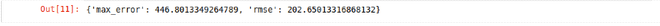Step 7: Visualizing the Polynomial Regression results using scatter plot and line plot of the input data and the predicted result.

## Python3

 `plt.scatter(data_points[``'x1'``], data_points[``'y'``])``plt.plot(data_points[``'x1'``], data_points[``'predicted_y'``])``plt.show()`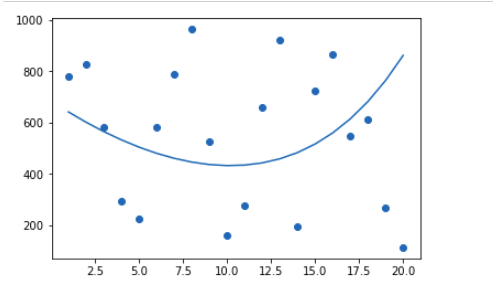My Personal Notes arrow_drop_up